Next: Normalization Using Functional Dependencies Up: Relational Database Design Previous: Representation of Information

Decomposition

1. The previous example might seem to suggest that we should decompose schema as much as possible.

2. Consider a design where Lending-schema is decomposed into two schemas

Branch-customer-schema = (bname, bcity, assets, cname)

Customer-loan-schema = (cname, loan#, amount)

3. We construct our new relations from lending by:

branch-customer =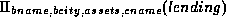customer-loan =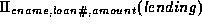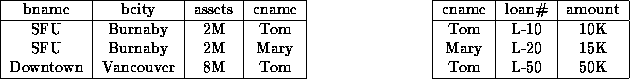Figure 7.2:   The decomposed lending relation.

4. It appears that we can reconstruct the lending relation by performing a natural join on the two new schemas.
5. Figure 7.3 shows what we get by computing branch-customercustomer-loan.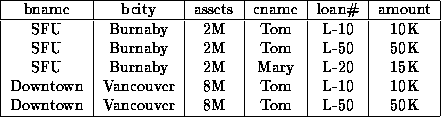Figure 7.3:   Join of the decomposed relations.

6. We notice that there are tuples in branch-customercustomer-loan that are not in lending.
7. How did this happen?
• The intersection of the two schemas is cname, so the natural join is made on the basis of equality in the cname.
• If two lendings are for the same customer, there will be four tuples in the natural join.
• Two of these tuples will be spurious - they will not appear in the original lending relation, and should not appear in the database.
• Although we have more tuples in the join, we have less information.
• Because of this, we call this a lossy or lossy-join decomposition.
• A decomposition that is not lossy-join is called a lossless-join decomposition.
• The only way we could make a connection between branch-customer and customer-loan was through cname.
8. When we decomposed Lending-schema into Branch-schema and Loan-info-schema, we will not have a similar problem. Why not?

Branch-schema = (bname, bcity, assets)

Branch-loan-schema = (bname, cname, loan#, amount)

• The only way we could represent a relationship between tuples in the two relations is through bname.
• This will not cause problems.
• For a given branch name, there is exactly one assets value and branch city.
9. For a given branch name, there is exactly one assets value and exactly one bcity; whereas a similar statement associated with a loan depends on the customer, not on the amount of the loan (which is not unique).
10. We'll make a more formal definition of lossless-join:
• Let R be a relation schema.
• A set of relation schemas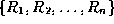is a decomposition of R if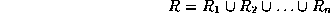• That is, every attribute in R appears in at least one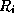for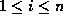.
• Let r be a relation on R, and let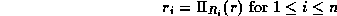• That is,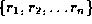is the database that results from decomposing R into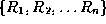.
• It is always the case that: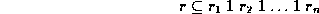• To see why this is, consider a tuple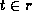.
• When we compute the relations, the tuple t gives rise to one tuple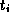in each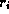.
• These n tuples combine together to regenerate t when we compute the natural join of the.
• Thus every tuple in r appears in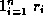.
• However, in general,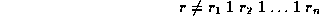• We saw an example of this inequality in our decomposition of lending into branch-customer and customer-loan.
• In order to have a lossless-join decomposition, we need to impose some constraints on the set of possible relations.
• Let C represent a set of constraints on the database.
• A decompositionof a relation schema R is a lossless-join decomposition for R if, for all relations r on schema R that are legal under C: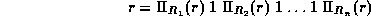11. In other words, a lossless-join decomposition is one in which, for any legal relation r, if we decompose r and then ``recompose'' r, we get what we started with - no more and no less.Next: Normalization Using Functional Dependencies Up: Relational Database Design Previous: Representation of Information

Osmar Zaiane
Thu Jun 18 12:56:34 PDT 1998